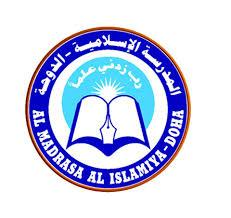# Annual Exam 2020 - Trial Version

15 Questions | Total Attempts: 1179SettingsAl Madrasa Al Islamiya - Doha

• 1.
Choose the correct answer النهر ....... الجسر
• A.

تحت

• B.

فوق

• C.

وراء

• D.

امام

• 2.
يطير العصفور ....... البيت
• A.

امام

• B.

تحت

• C.

فوق

• D.

شمال

• 3.
لعلم الهند ................... الوان
• A.

ثلاث

• B.

ثلاثة

• 4.
تقف البنت .................. المرآة
• A.

فوق

• B.

امام

• 5.
............  للديك رجلان
• A.

اثنان

• B.

اثنتان

• 6.
يسأل المدرس ....... الدرس
• A.

إلى

• B.

عن

• C.

في

• D.

ب

• 7.
ينظف الإبن ................ المكنسة
• A.

الى

• B.

عن

• C.

في

• D.

ب

• 8.
يذهب جحا ................... السوق
• A.

الى

• B.

عن

• C.

في

• D.

ب

• 9.
السمك .................. الماء
• A.

الى

• B.

عن

• C.

في

• D.

ب

• 10.
Select true or false للفيل ثلاث ارجل
• A.

True

• B.

False

• 11.
البقرة حيوان داجن
• A.

True

• B.

False

• 12.
ولدان واحد
• A.

True

• B.

False

• 13.
• A.

يوم الاحد

• B.

يوم الجمعة

• C.

يوم السبت

• D.

يوم الإثنين

• 14.
Sunday
• A.

يوم الإثنين

• B.

يوم الثلاثاء

• C.

يوم الأحد

• D.

يوم الأربعاء

• 15.
Saturday
• A.

يوم الأربعاء

• B.

يوم الجمعة

• C.

يوم الثلاثاء

• D.

يوم السبت

Related TopicsBack to top